×

Search anything:

# Understand different types of Boosting Algorithms

#### Machine Learning (ML)Boosting is an algorithm aimed to ﬁt a meta-model (a model ﬁtted on the results of other models, usually called weak learners) on data in a greedy fashion.
In this article we will dive deep into understanding Boosting and then we are going to see rapidly some derived algorithms that is the types of Boosting algorithms such as:

• XGBoost
• LightGBM

Some of these have been used in recent years to obtain great performances in Kaggle competitions!

# How Boosting works?

Given the definition above, the algorithm works in a way that ﬁt weak learners on weighted versions of the data, where more weight is given to the misclassiﬁed observation in earlier rounds of the algorithm (greedy). The weak learners can be any classiﬁcation or regression algorithm. Per deﬁnition they are very resistant to overﬁtting, because the objective of this technique is to obtain best performance on an extended ”image” of the data (great power of generalization) as is an ensemble of smaller ”images” (weak learners).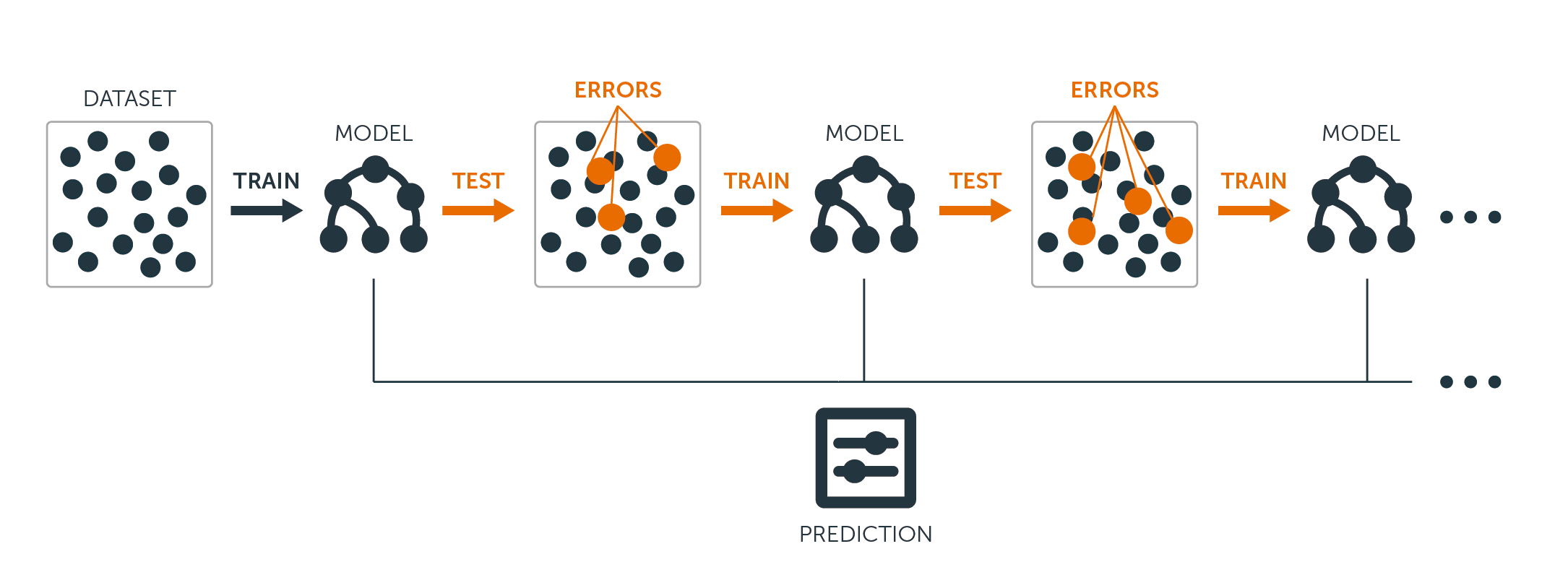Beginning from this simple concept, let's see how the other algorithms developed upon this.

The basis of this algorithm is the Boosting main core: give more weight to the misclassified observations.
In particular, AdaBoost stands for Adaptive Boosting, meaning that the meta-learner adapts based upon the results of the weak classifiers, giving more weight to the misclassified observations of the last weak learner (usually a decision stump : one level decision trees, meaning that the tree is made based upon one decision variable and it's composed by a root and all the childs directly connected to the root)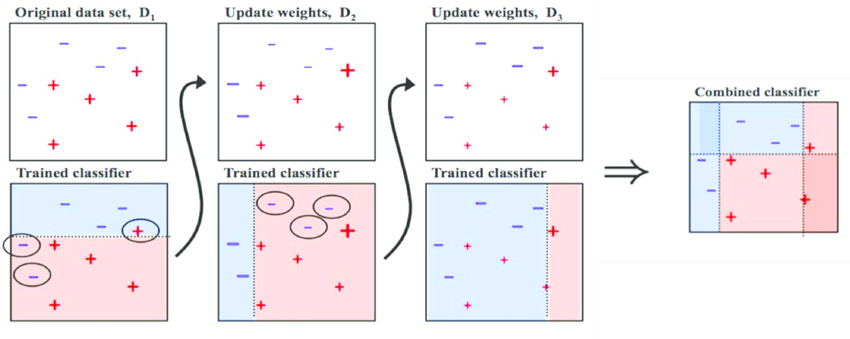Notice the circled + and - signs: They are growing if misclassified, otherwise they reduce if correctly classified.
And here we can see the meta-learner general form: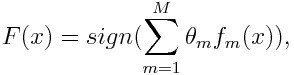Where f corresponds to the weak learner and theta stands for the weight associated to the specific weak classifier

With Gradient Boosting we have a generalization of boosting techniques in which is possible to optimize the meta-learner based on an arbitrary differentiable loss function. What does it mean?

It means that changes everything! As we have seen in AdaBoost, the meta-learner is optimized based on the misclassified observations by the weak learners, in Gradient Boosting the meta-learner is optimized (with tecniques like the almighty gradient descent) based upon a loss function. Like this one (the least square loss function):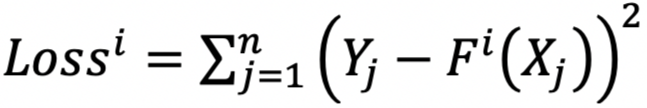Where Y is the real value of the target variable and F(X) is the prediction of the meta-learner function, based on the values of the variables associated to the evaluated target value Y.

# XGBoost

Here we have one of the most used Boosting algortim (if not the most used). It has gained his fame through Kaggle competitions and it's winners, thanks to the fine tuning (and ensembling) of these algorithms.

The majors benefits of XGBoost are:

• Speed
• High scalability
• Parallelization
• Usually overperform other algorithms

# Why XGBoost so efficient?

XGBoost stands for eXtreme Gradient Boosting, meaning that it was projected with the purpose to increase speed and performances of the Gradient Boosting algorithms. In particular it uses trees as weak-learners and it optimize the weak-learners construction (thanks to leaf-wise tree growth strategy and to histogram based tecniques, for the evaluation of the most profitable tree branch split) to make the algorithm memory and speed effcient. Moreover it helps with performance because (as advised by Tianqi Chen, the library developer), when we use the regularized model formulation, we obtain major performance tweaks, because regularization reduce sensibly overfitting.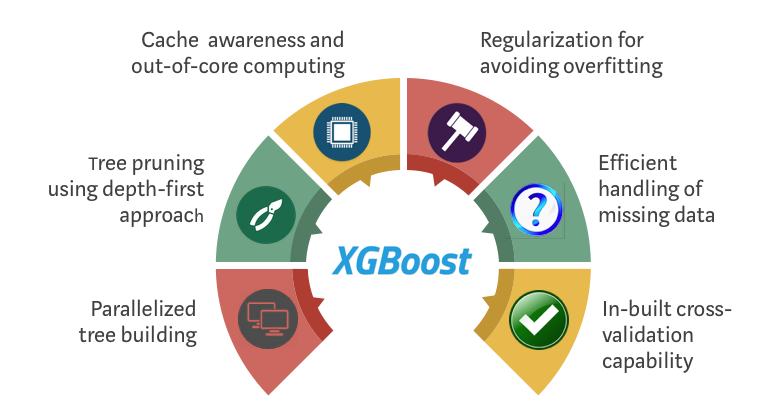in the picture above we have some of the major advantages of XGBoost.
More theoretical information can be found here

# LightGBM

LightGBM takes XGBoost and make it more faster thanks to a couple of improvements in speed given by:

• GOSS: Gradient-based One Side Sampling, an algorithm that excludes low-gradient data instances, to reduce the number of observations.
• EFB: Exclusive Feature Bundling, which bundle mutually exclusive features, in order to reduce the number of features.

Thanks to these two algorithmical tweaks we have a more speed and memory efficient Gradient Boosting algorithm (one of the most efficient out there, if not the most efficient).

# Conclusion

Now that we have seen how Boosting works and some derived algorithms, you can dig deeper in the specific algorithms to see which is your favourite and/or most suitable for your needs.